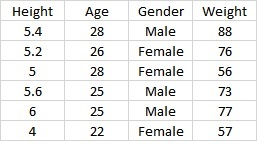top of pageSearch

# Gradient Boost for Regression Explained

Gradient boost is a machine learning algorithm which works on the ensemble technique called 'Boosting'. Like other boosting models, Gradient boost sequentially combines many weak learners to form a strong learner. Typically Gradient boost uses decision trees as weak learners.

Gradient boost is one of the most powerful techniques for building predictive models for both classification and Regression problems. In this blog we will see how Gradient boost works with Regression.### What is Boosting?

Boosting idea is to train weak learners sequentially, each trying to correct its predecessor. This means, the algorithm is always going to learn something which is not completely accurate but a small step in the right direction. As the algorithm moves forward by sequentially correcting the previous errors, it improves the prediction power.To understand the Gradient boost below are the steps involved. In Gradient boosting weak learners are decision trees.

Step1: Construct a base tree with single root node. It is the initial guess for all the samples.

Step2: Build a tree from errors of the previous tree.

Step3: Scale the tree by learning rate (value between 0 and 1). This learning rate determines the contribution of the tree in the prediction

Step4: Combine the new tree with all the previous trees to predict the result and repeat step 2 until maximum number of trees is achieved or until the new trees don't improve the fit.

The final prediction model is the combination of all the trees.### Regression Example

To understand how Gradient boost works, lets go through a simple example.

Suppose we have below table of sample data with Height, Age and Gender as input variables and weight as the output variable.To predict the weights, step1 is to create a tree with root node. For the initial guess, we can use average, mean squared error, mean absolute error etc.,

If we assume that the average of weights of all the samples as our initial guess then 71.2 (88+76+56+73+77+57/6=71.2) would be our initial root node.Step2 is to build a tree based on errors from previous tree. The errors that the previous tree made is the difference between the Actual weight and the predicted weight. This difference is called Pseudo Residual.Now we build a tree with maximum leaf nodes as 4 using Height, Age and Gender to predict the residuals(Error). If more that 1 weight fall on the same leaf, then we take the average of the weights as the leaf node.Step 3 is scaling tree with learning rate. Assuming the learning rate as 0.1.

Step 4 is combining the trees to make the new prediction. So, we start with initial prediction 71.2 and run the sample data down the new tree and sum them.If we observe the new predicted weights, we can see a small improvement in the result compared to the average weight from initial assumption. To further improve the result, we repeat the steps 2 and 3 and build another tree from the new pseudo residuals to predict the weights.Again build a new tree with the new pseudo residuals.Now we combine the new tree with all the previous trees to predict the new weights. So, we start with initial prediction and sum it with scaled result of 1st tree and then sum with scaled result of new tree.From the new predicted weight, we can observe there is further improvement in the result. Again we calculate the pseudo weights and build new tree in the similar way. These steps are repeated several times until the new tree doesn't decrease the pseudo residual value or till maximum number of trees are built.

So the final predicted model would beNow if we get new data for test, we pass it through the above model and calculate the weight of the person.

### Conclusion

Gradient boost is a powerful boosting technique. It improves the accuracy of the model by sequentially combining weak trees to form a strong tree. In this way it achieves low bias and low variance.